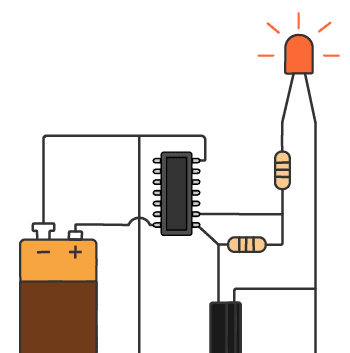# Basic Electronics Resource Library## Current Limiting Resistor

A current limiting resistor is a resistor that is used to reduce the current in a circuit. A simple example is a resistor in series with an LED. You would usually want to have a current limiting resistor in series with your LED so that you can control the amount of current through the LED. […]

## Voltage Divider Circuits Give You Power Over The Voltage

A voltage divider is a circuit that divides a voltage between two resistors. It’s extremely useful to know! If you know how the voltage divider works you can easily make sensor circuits or calculate values in a more complex circuit.

## Understanding Basic Electronics is Easy

Yes, basic electronics is easy. As long as you don’t make it complicated ;) An electrical current is the flow of electrons in a wire. Electrons flow when you have a “closed loop” – a path from the negative to the positive terminal of a battery. For example if you connect a small light bulb […]

## Series and Parallel Circuits

In electronics we can find both series and parallel circuits. A series circuit is a circuit where the components are connected in a consecutive chain. This gives the current only one path to take. A parallel circuit is a circuit where the components are connected parallel to each other. So the current will flow in […]

## The Thevenin Theorem – A Simple Explanation

The Thevenin Theorem explains how to simplify a complex circuit. It states that any linear circuit with only voltage sources, current sources and resistors can be simplified to a voltage source with a series resistor. But what does this really mean? Sometimes a picture says more than thousand words, so here are two pictures to […]

## Basic Electronic Components Used in Circuits

I learned about the basic electronic components by going to the library and reading books. I was just starting out. And I felt like a lot of the books explained everything in a difficult way. In this article, I will give you a simple overview, with an explanation of the basic electronic components – what […]